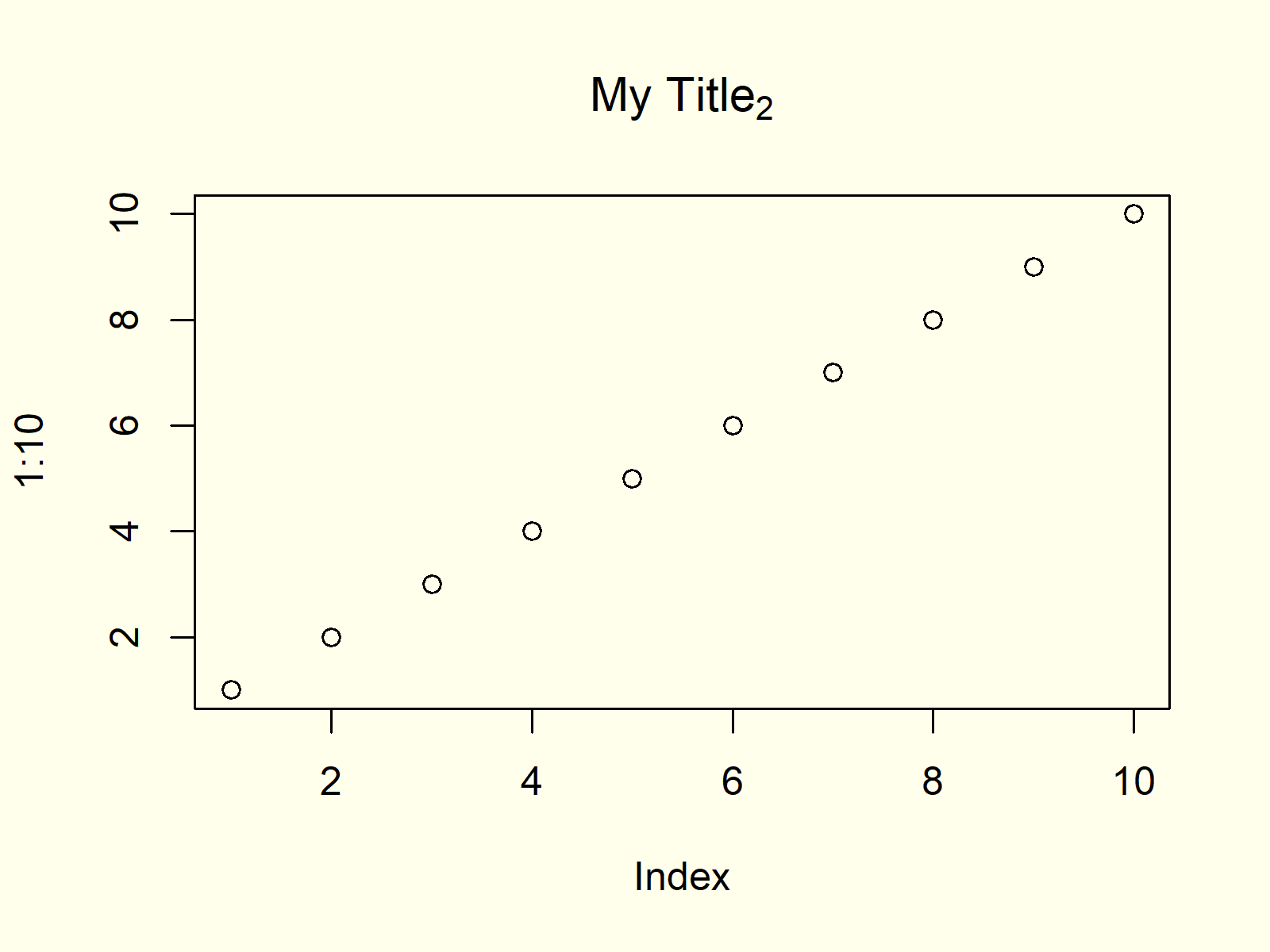# Add Subscript and Superscript to Plot in R (3 Examples)

In this tutorial, I’ll explain how to create a subscript or superscript in R.

The tutorial consists of three examples for the addition of subscripts and superscripts. To be more specific, the article will contain these topics:

Let’s take a look at some R codes in action.

## Example 1: Adding Superscript to Plot

Example 1 shows how to add a superscript (i.e. the power of 2) to a text in a plot in R.

For this task, you need to apply the expression function and add the ^ symbol and the value you want to show as a superscript behind the character string containing the title of your plot. Have a look at the following R code and the resulting plot:

`plot(1:10, main = expression("My Title"^2)) # Add superscript to plot`Figure 1: R Plot with Superscript in Main Title.

Figure 1 shows the output of the previous R syntax. As you can see, the main title of the plot contains a superscript.

Note that you may add a superscript to any other kind of text (e.g. axis labels or text within a graph) in a Base R graphic.

## Example 2: Adding Subscript to Plot

In Example 2, I’ll show how to add a subscript to a plot title. For this task, we also need to use the expression function of the programming language. In contrast to Example 1, we need to wrap square brackets (i.e. ) around the value we want to show as a subscript:

`plot(1:10, main = expression("My Title")) # Add subscript to plot`Figure 2: R Plot with Subscript in Main Title.

As you can see in Figure 2, our plot title contains a subscript.

## Example 3: Adding Multiple Superscripts & Subscripts to Plot

It is also possible to add several superscripts and subscripts to a text element using the expression function and the symbols ^ and [] as shown in the previous examples. Note that you need to add the star symbol (i.e. *) after a superscript or subscript, in case you want to continue with text afterwards. Have a look at the following example code:

`plot(1:10, main = expression("My"^1*"Long"*"Title"["b"]^"a")) # Multiple numbers`Figure 3: R Plot with Superscript & Subscript in Main Title.

We added multiple superscripts and subscripts. Some of them are numbers and others are letters.

## Video, Further Resources & Summary

Do you need further information on the examples of this article? Then I can recommend watching the following video of my YouTube channel. In the video, I’m explaining the content of this tutorial.

Please accept YouTube cookies to play this video. By accepting you will be accessing content from YouTube, a service provided by an external third party.If you accept this notice, your choice will be saved and the page will refresh.

Furthermore, you might have a look at the related tutorials of this website:

This article illustrated how to include superscripts and subscripts on axis or title text of a plot in the R programming language.

In the examples of this R programming tutorial, I have shown how to add subscripts and superscripts to Base R plots. Please note that the expression function could also be used in other graphical environments such as the ggplot2 package. Furthermore, you may use the expression function to add exponents to mathematical equations.